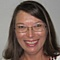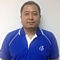# Business Value Metrics### Recent Posts

The Trust Equation

Communication in Co-located & Distributed Agile Teams

Trust: The most betrayed fellow in Scrum

Good recipes to fail in Scrum

In Agile and Scrum, business justification is continuously verified and assessed. A project should make sense in all phases of its life cycle, and it's important to keep assessing the business value in in agile projects. It also helps in deciding whether to keep the project running or to terminate/close it. The business value of an investment is usually assessed in the financial terms.

While reading about Agile and Scrum, we run across terms such as ROI, NPV, and IRR, but we are only made acquainted with these concepts theoretically, not quantitatively. In this article I have provided a few methods by which business value is calculated. I hope they can help those of us who are working in Agile environments or projects.

The following are the quantitative methods used to determine the business value of a project:

1. Return on investment (ROI)
2. Internal rate of return (IRR)
3. Net present value (NPV)

### Return on investment (ROI)

To calculate the ROI, we take the gain from an investment and subtract the cost of the investment. Then, divide the total by the cost of the investment as follows:

ROI = (Gains – Cost)/Cost

It is a simple calculation that indicates the bottom line of the return on any investment. ROI can be used as a starting point for sizing up any investment. One major factor that is not considered in an ROI calculation is time. The NPV and IRR equations below consider the time value of money.

### Net present value (NPV)

The value of a dollar today and the value of a dollar one year from today are not the same. If the market interest rate for the year is r, then we multiply by the interest rate factor to move the cash inflow from the beginning to the end of the year. This process of moving the value or cash inflow forward is known as compounding.

The formula for calculating the future value of cash inflow:

FV = C x (1+r)^n

where FV = Future Value, C = Cash flow, r = interest rate, and n = time.

Now suppose we want to calculate today's value of \$1,000, which we expect to receive one year from today. We would have to move the cash inflow backward in time. Thus, the process of moving the cash inflow backward in time, i.e., finding the equivalent value today of a future cash inflow, is known as discounting.

The formula for calculating the present value of cash inflow:

PV = C / (1+r)^n

where PV = Present Value, C = Cash flow, r = interest rate, and n = time.

### Internal rate of return (IRR)

If we know the present value and cash flows of any investment opportunity, but we don't know the interest rate that equates them, the interest rate is called the internal rate of return (IRR). IRR is also the interest rate that sets the net present value of cash flow for a particular project equal to zero.

Here is an example to help you understand IRR: Suppose you have an investment opportunity that requires a \$1,000 investment today that will pay off in \$2,000 in six years.

In this scenario, we will need to find the interest rate (say r) at which the NPV of the investment is zero by solving the following equation:

NPV = -1000 + 2000/(1+r)^6 = 0

Solving further:

1000 x (1+r)^6 = 2000

1+r = (2000/1000)^?

1+r = 1.1225

Here r can also be called the interest rate you would need to earn on \$1000 to reach a future value of \$2000 in six years.

On solving the equation, we get r = 12.25.

Here, r is the IRR of this investment opportunity.

These methods are only a quantified way of calculating the business value for a project. There are various others that are not discussed in this article, which also can help in determining the business value for a project.

Reference
Berk, Jonathan and Peter DeMarzo and Ashok Thampy. Financial Management. Pearson Education, 2010.

Posted on: April 25, 2016 05:26 AM | Permalink

Please login or join to subscribe to this itemNetwork:357I enjoyed the article.Network:713thanks for the formula :)Network:4306Thanks Judy & Ahmad for your valuable feedback and comments.